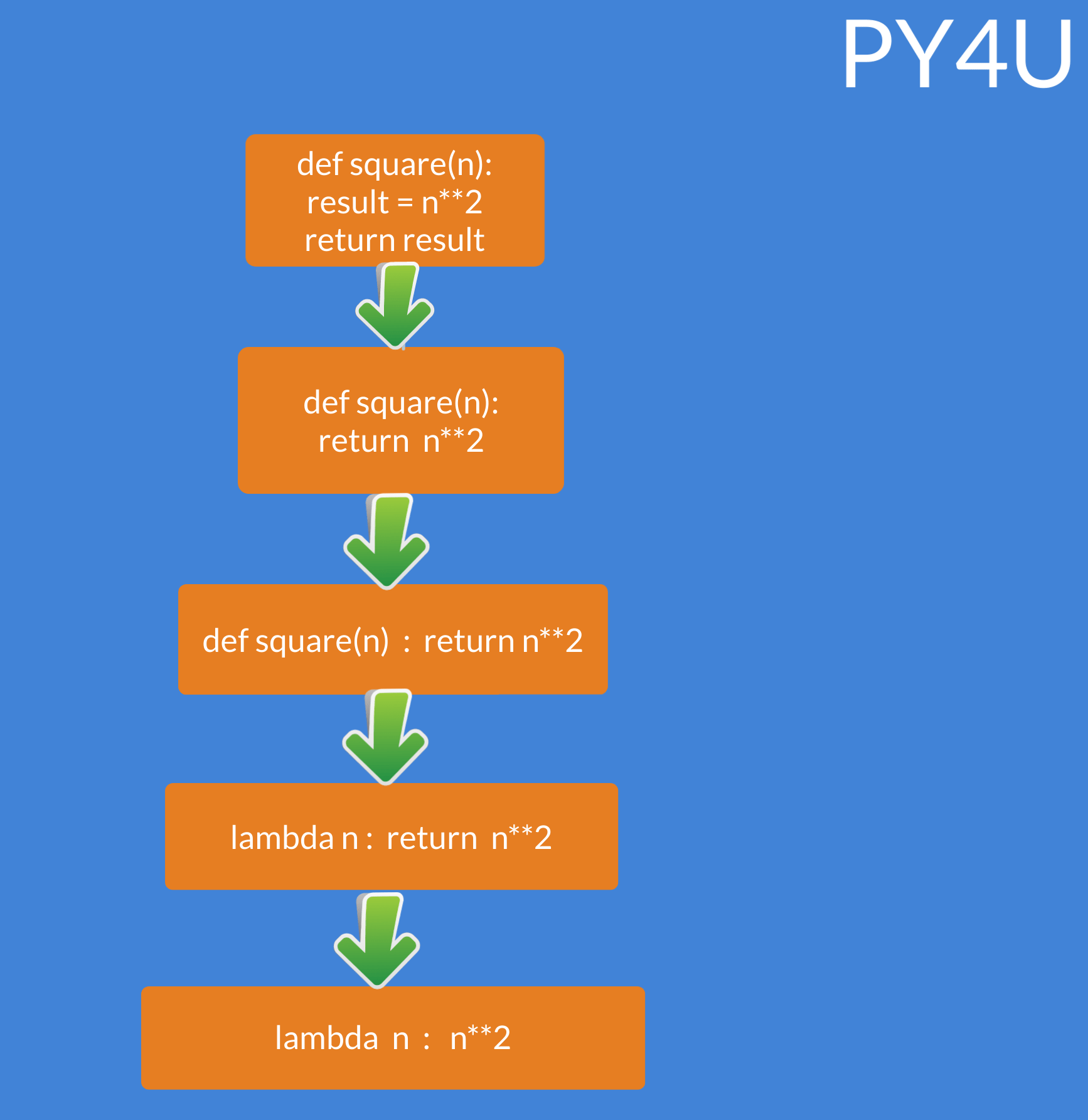Map Function And Lambda Expressions

map() function:

Remember when we were taking multiple space-separated numbers( in form of string ) as input and casting them to be a integer ? This was the first time we used map function. We were doing something like this :

• list(map(int,input().split()))

But we actually didn't discuss about the map function back then. So what really is map function? Well most of the times map functions are used on a list where every element of a list undergoes some operation. While taking input , We were using the map function to convert numbers( datatype of string ) to integers and often you'll be using it with functions as well. Let us see an example on this.

def square(n):
return n**2

my_list=[1,2,3,4,5,6,7,8]
print(map( square , my_list ))
print(list(map(square,my_list)))
output:
< map object at 0x7fdc6b9a44a8>
[1, 4, 9, 16, 25, 36, 49, 64]Inorder for the map function to yield some useful results , it is important to cast it into a list else it will output the location where the map object is stored at in the memory.Notice how i didn't invoke the square function ( no parenthesis ) when passing it to map function. This is because the map function will invoke the square function for us so we actually don't need to invoke the square function ourselves.lambda expression

Lamda expressions are simple one line functions . They are also called anonymous functions because they don't have any name assigned to them. Also you do not need to specify the return keyword inside the lambda function since it is automatically assumed there. We also don't use the def keyword , rather we use lambda. Let us look at its syntax.

• lambda parameter_name : # some code

Below i have explained how to convert a normal function to a lambda function.Note that lambda functions can be assigned to a variable however generally we don't do that. Rather we use lamda functions in combination with other functions.

fivetimes = lambda n : 5*n
print(fivetimes(5))
output:
25

let us use lambda function with map function. In the code below i have a list of integers and i wish to cube them using map function.

print(list(map(lambda n : n**3,[1,2,3,4,5,6,7])))
output:
[1, 8, 27, 64, 125, 216, 343]

Popular Tutorials